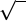Study Guides (400,000)
US (230,000)
UC-Irvine (3,000)
CHEM (100)
Quiz

# CHEM 132B Study Guide - Quiz Guide: Haplogroup R2A, Term Symbol, Magnetic Quantum NumberExam

Department
Chemistry
Course Code
CHEM 132B
Professor
Eric Potma
Study Guide
Quiz

This preview shows half of the first page. to view the full 3 pages of the document.Chem 132B Practice Quiz 2 Key
Version A
Problem 1
Indicate whether the following statements are true or false.
a) hb
l2,b
lyi= 0 True.
b) hb
l2
z,b
lyi= 0 False.
c) b
l2and b
l2
zshare the same set of eigenfunctions. True.
d) If the magnitude of the orbital angular momentum is h, then there is
also a projection ±honto the z-axis. False.
Problem 2
Indicate whether the following statements are true or false.
a) Spin-orbit coupling is a negligible eﬀect in all atoms. False.
b) In the Clebsch-Gordan series, the total angular momenta jof individual
electrons are combined to form the combined total angular momentum J.
False.
c) When the spin angular moment of an electron couples with its orbital
angular moment, states that were initially degenerate are split, which
implies that now the number of states has increased due to spin-orbit
coupling. False.
d) We can both know the magnitude of the total angular momentum and its
projections mjalong the z-axis. True.
1